Browse by Topic
Related Topics

# Mathematica Q&A Series: Converting to Conventional Mathematical Typesetting

Got questions about Mathematica? The Wolfram Blog has answers! We’ll regularly answer selected questions from users around the web. You can submit your question directly to the Q&A Team.

This week’s question comes from Jee:

How can I transform the output of partial differentiation such as f(1, 0)[x, y] to the mathematical form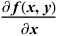?

Read below or watch this screencast for the answer (we recommend viewing it in full-screen mode):

We will assume that the reader is already familiar with the basics of differentiation in Mathematica. To quickly catch up with the topic, one should read the recent Q&A blog post “Three Functions for Computing Derivatives”.

The typesetting in which derivatives are displayed in Mathematica may vary depending on the situation. Yet they are all interpreted through the function Derivative, which is an equivalent of the differential operator in traditional mathematics. Take a look at the following list, where each element represents a different way differentiation can be entered: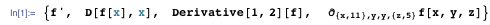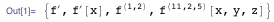For the last element we used the key sequence Esc + d + t + Esc. Despite different appearances, the underlying expressions are of the same syntax, which can be revealed with the function InputForm: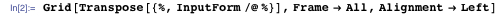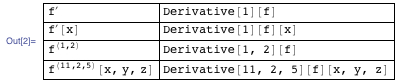If one deals with differentiation of undefined functions, the output will always be of the form shown in the left column, dictated by the underlying syntax of the right column. How does one go from this typesetting to other conventional mathematical notations? Well, we can start by using the function TraditionalForm, which was created exactly for this purpose: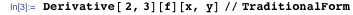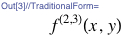This is a type of traditional mathematical notation where partial derivatives are denoted by indexes in the function’s superscript. Yet this is not what Jee is looking for, which is a convention that uses the symbol ∂. The full list of Mathematica syntax that may be displayed via TraditionalForm as a conventional notation can be found in the extensive tables of the tutorial TraditionalForm Reference Information. We can learn from it that the notation we need can be provided by the function D: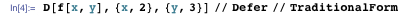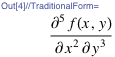The function Defer was used to prevent Mathematica from automatic evaluation from the syntax of function D to the one of function Derivative. Great, but what if after some derivations we end up with an answer given in terms of indexed notation? How do we display it in terms of the symbol ∂? Well, we can construct a function that reads out the elements of syntax given by Derivative, puts them in the form of D, and applies TraditionalForm: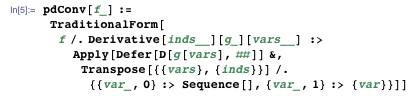To better understand the structure of the function, consider the table below.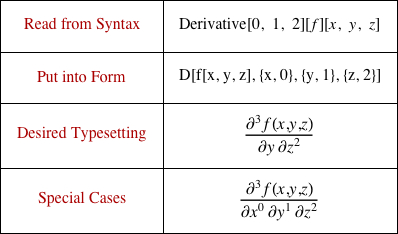In the particular case considered, pdConv will read indexes 0, 1, and 2; variables x, y, and z; and function name f from the syntax provided by Derivative and put them in the syntax of function D. The function pdConv will then use Defer and TraditionalForm to display the result in desired conventional typesetting. Note that zero- and first-order derivatives are “Special Cases” labeled in the last row. They should be displayed differently compared to higher-order derivatives, and are handled in the last line of pdConv.

Function pdConv will now work with any properly defined expression. Here is a computation that ends up displayed according to the underlying Derivative syntax: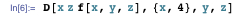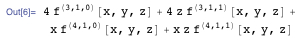Applying our function pdConv will typeset this expression in terms of the symbol ∂: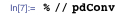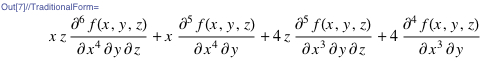After we copy and evaluate the output cell, the Defer function used inside pdConv will release its hold on the evaluation: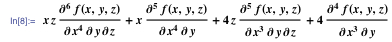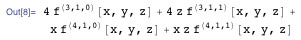And so this expression is still computable. For example an explicit function can be substituted instead of f(x, y, z) for further evaluation: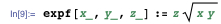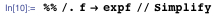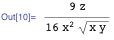Hence Mathematica allows computing with expressions in traditional mathematical typesetting if they are properly defined, unlike many other systems that only help to format and display the typesetting. The function pdConv acts simply as TraditionalForm with respect to any other part of an expression that is not related to Derivative: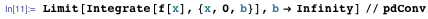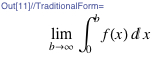If you have a question you’d like answered in this blog, you can submit it to the Q&A Team. For daily bite-sized Mathematica tips, follow our @MathematicaTip Twitter feed.